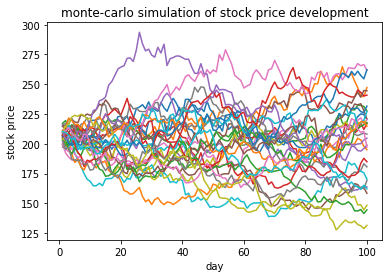# Monte-carlo simulation in PythonIn one of my posts I have introduced the concept of random walk forecasting, using Python for implementation. In this post I want to conduct a monte-carlo simulation in Python. More specifically, I will use monte-carlo simulation in Python to assess risks associated with stock price volatility.

## Setting up data for monte-carlo simulation in Python

For this I will use a the following fictional stock price history:

```# declare list with fictional daily stock closing prices
history_prices = [180,192,193,195,191,199,198,200,199,203,205,207,205,208,201,203,204,201,205,206,207]
print(stockPrices)
```
```[180, 192, 193, 195, 206, 211, 191, 204, 215, 190, 205, 207, 205, 211, 222, 215, 245, 201, 205, 206, 214]
```

I now proceed to importing relevant modules. Most importantly I need to use a module for visualizing the random walks and simulation. And, I need a module for generating random numbers.

## Modules for monte-carlo simulation in Python

In preparation of next steps I will now import all relevant Python modules:

```# import statistics for calculating e.g. standard deviation of price history
import statistics as stat
# import pyplot for plotting
import matplotlib.pyplot as plt
# import random for random number generations
import random as rnd
```

Assuming random stock price movement I derive standard deviation from relative changes in fictional price history such that I will be be able to model random stock price movements:

```relative_prices = []
for i in range(0,len(history_prices)):
if i == 0:
pass
else:
relative_prices.append((history_prices[i]-history_prices[i-1])/(history_prices[i-1]))
std_prices = stat.stdev(relative_prices)
print(std_prices)
```
```0.021375589655016836
```

Having modules and statistics in place I now model a random walk. I will need random walks for conducting the monte-carlo simulation in Python.

## Modeling a random walk as part of the simulation

Now I model one exemplaric random price walk for 100 days into the future, assuming random price movement based on the standard deviation of random prices. I assume a random normal distribution. The last known price in history serves as starting point:

```# modeling a random price walk over 100 days
# -- conduct calculation, define function
def randomWalk(stdev,pastPrices):
days = [i for i in range(1,101)]
prices = []
price = pastPrices[-1]
for i in range(1,101):
price = price + price*rnd.normalvariate(0,stdev)
prices.append(price)
return([days,prices])
# -- conduct calculation, use function
prices = randomWalk(std_prices,history_prices)
# -- visualize random walk in a line plot
plt.plot(prices,prices)
plt.title("random price walk")
plt.xlabel("day")
plt.ylabel("stock price")
```
`Text(0, 0.5, 'stock price')`

I successfully implemented and visualized a random walk. A random walk represents one possible price development trajectory. The random walk is based on historic probability, i.e. historic data. If I conduct several random walks then I can make a statement about the risk associated with the stock. Several random walks together form a monte-carlo simulation. In the next section I will implement a monte-carlo simulation in Python using above random walk model.

## Implementing the simulation model

I can repeat this process, by re-calculating additional random walks, thereby creating a monte-carlo simulation of stock price movements. In below example I repeat the random walk process for 30 separate walks:

```plt.figure()
for i in range(0,30):
prices = randomWalk(std_prices,history_prices)
plt.plot(prices,prices)
plt.title("monte-carlo simulation of stock price development")
plt.xlabel("day")
plt.ylabel("stock price")
```
`Text(0, 0.5, 'stock price')`

## Conclusion and relevant references

In this article I introduced the concept of a monte-carlo simulation. I used random walks of stock price developments to illustrate this. A stock price can be modeled as a random walk, and a monte-carlo simulation is a repetition of several random walks. Such as simulation can be used to evaluate risks associated with stock price volatility.

I have written several other related articles. I list them and other articles below. I recommend them to anyone interested in monte-carlo simulation.